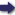(London :  Kegan Paul, Trench, Trübner & Co.,  1910.)

 Tools

## Search this bookPrev Page 195 Next``` CHAPTER LXXVIII. 195 If vou want to know the karctnas, first determine the Rule how to f> -1 fill ^^'^ ^'^® lunar days, and find out m what part of them the date karanas. in question falls, which is done in this way:— Subtract the corrected place of the sun from the corrected place of the moon. The remainder is the distance between them. If it is less than six zodiacal signs, the date falls in the white half of the month ; if it is more, it falls in the black half. Reduce this number to minutes, and divide the pro¬ duct by 720. The quotient represents tithis, i.e. com¬ plete lunar days. If you get by the division a re¬ mainder, multiply it by 60 and divide the product by the mean bhukti. The quotient represents ghatis and minor fractions, i.e. that portion of the current day which has already elapsed. This is the method of the canones of the Hindus. The distance between the corrected places of sun and moon must be divided by the mean bhiddi. This, how¬ ever, is impossible for many of the days. Therefore they divide this distance by the difference between the daily revolutions of sun and moon, which they reckon for the moon as 13 degrees, for the sun as i degree. It is a favourite method in rules of this kind, especi¬ ally in Indian ones, to reckon by the mean motion of sun arid moon. The mean motion of the sun is sub¬ tracted from the mean motion of the moon, and the remainder is divided by 732, which is the difference between their two middle hhuktis. The quotient then represents days and ghatis. The word buht is of Indian origin. In the Indian Expiana- language it is bhukti ( = the daily motion of a planet), hhukti. If the corrected motion is meant, it is called bhukti sphuta. If the mean motion is meant, it is called bhukti mctdhyamct, and if the buht which renders equal is meant, it is called bhuktyantarct, i.e. the difference between the two bhuktis. ```Prev Page 195 Next